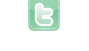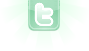This site requires JavaScript, please enable it in your browser!Wrong Answer to a problem?

I wanted to do a problem(math) in greenfoot with this code:
public int getRemainder(int i, int i2)
{
double i3 = (i / i2);
i3 = i3 * 100;
int i33 = (int) i3;
return i33;
}
Unfortunately, when inputted with values of 40 and 100, it gives 0. Why is this happening? Note: I tried this with a calculator. It gives 40
When you do i / i2 in the first line of the method, you are dividing an int by an int, which is therefore an integer division. 40/100 is 0 (integer division rounds down). So then the end result is zero. If you add a cast (the numerator is enough) to double, then you will do a double division, resulting in 0.4 (or rather, the nearest number representable: 0.4 cannot be exactly represented in binary), and it will all work out. Long story short, this should fix it:
double i3 = ((double)i / i2);
Another way to handle this is simply
public int getRemainder(int i, int i2)
{
return (i % i2);
}
This will always return the remainder portion of the division. As short as this is, dropping the method and using (i % i2) in the calling method might be preferable.
You need to login to post a reply.